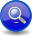#Boost C++ Libraries

...one of the most highly regarded and expertly designed C++ library projects in the world.

##### Kleene (`*a`)
###### Description

The kleene operator, `*a`, is a unary operator that matches its operand zero or more times.

```// forwards to <boost/spirit/home/qi/operator/kleene.hpp>
#include <boost/spirit/include/qi_kleene.hpp>
```

Also, see Include Structure.

Notation

`a`
###### Expression Semantics

Semantics of an expression is defined only where it differs from, or is not defined in `UnaryParser`.

Expression

Semantics

`*a`

Match `a` zero or more times.

###### Attributes

Expression

Attribute

`*a`

```a: A --> *a: vector<A>
a: Unused --> *a: Unused```

###### Complexity

The overall complexity of the Kleene star is defined by the complexity of its subject, `a`, multiplied by the number of repetitions. The complexity of the Kleene star itself is O(N), where N is the number successful repetitions.

###### Example
NoteThe test harness for the example(s) below is presented in the Basics Examples section.

Some using declarations:

`using boost::spirit::qi::int_;`

Parse a comma separated list of numbers and put them in a vector:

```std::vector<int> attr;
test_phrase_parser_attr(
"111, 222, 333, 444, 555", int_ >> *(',' >> int_), attr);
std::cout
<< attr << ',' << attr << ',' << attr << ','
<< attr << ',' << attr
<< std::endl;
```